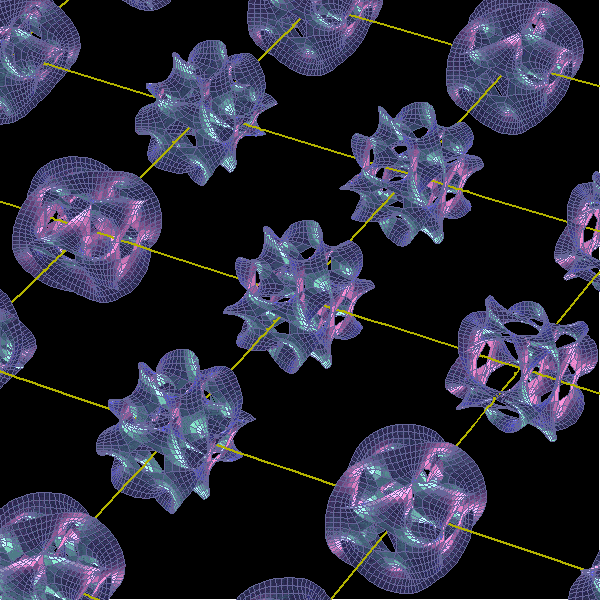# Science – Understanding A 10 Dimensional Universe

A summary of the proposed 10-dimensional universe and theory suggests at each. Full article is here: https://futurism.com/understanding-10-dimensional-universe/

1. First dimension – length
2. Second dimension – height
3. Third dimension – depth
4. Fourth dimension – time
5. Fifth dimension – we would see a world slightly different from our own that would give us a means of measuring the similarity and differences between our world and other possible ones.
6. Sixth dimension – we would see a plane of possible worlds, where we could compare and position all the possible universes that start with the same initial conditions as this one.
7. Seventh dimension – you have access to the possible worlds that start with different initial conditions.
8. Eighth dimension – again gives us a plane of such possible universe histories, each of which begins with different initial conditions and branches out infinitely.
9. Ninth dimension – we can compare all the possible universe histories, starting with all the different possible laws of physics and initial conditions.
10. Tenth and final dimension, we arrive at the point at which everything possible and imaginable is covered.Image credit: Berkeley Center for Cosmological Physics